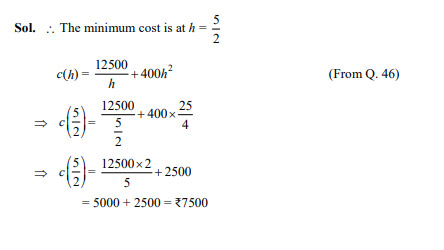﻿ Board Paper of Class 12 2022 Mathematics Term-1 Set-4 Solutions | Aasoka

# Board Paper of Class 12 2022 Mathematics Term-1 Set-4 CODE 065/2/4

#### General Instructions:

1. This question paper comprises 50 questions out of which 40 questions are to be attempted as per instructions. All questions carry equal marks.
2. The question paper consists of three Sections - Section A, B and C.
3. Section - A contains 20 questions. Attempt any 16 questions from Q. No. 1 to 20.
4. Section - B also contains 20 questions. Attempt any 16 questions from Q. No. 21 to 40.
5. Section - C contains 10 questions including one Case Study. Attempt any 8 from Q. No. 41 to 50.
6. There is only one correct option for every Multiple Choice Question (MCQ). Marks will not be awarded for answering more than one option.
7. There is no negative marking.
SECTION-A
(In this section, there are 20 questions. Any 16 are to be attempted).

## Differential of is

Ans. (a)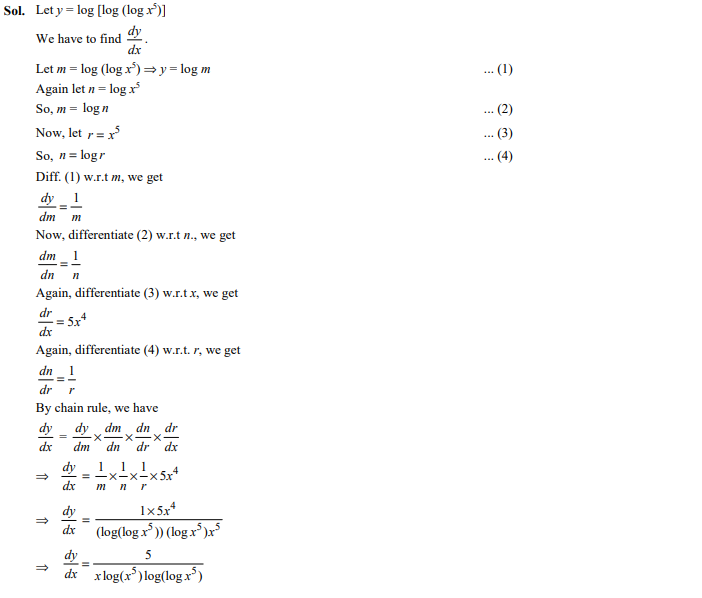## The number of all possible matrices of order 2 × 3 with each entry 1 or 2 is

1. 16
2. 6
3. 64
4. 24

Ans. (c)

Sol: Number of elements in matrix of order 2 × 3 = 6
Number of all possible matrices = = 64

## A function is defined as Then the function has

1. no minimum value
2. no maximum value
3. both maximum and minimum values
4. neither maximum value nor minimum value

Ans. (d)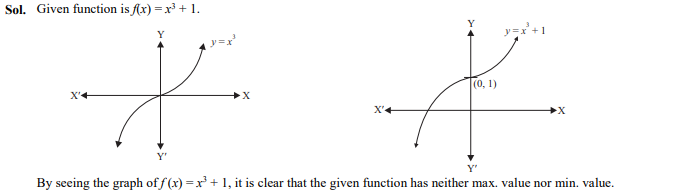## If then is

Ans.(a)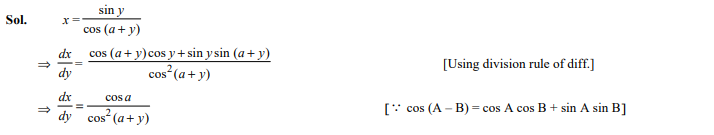## The points on the curve where tangent is parallel to x-axis are

1. (±5, 0)
2. (0, ±5)
3. (0, ±3)
4. (±3, 0)

Ans. (b)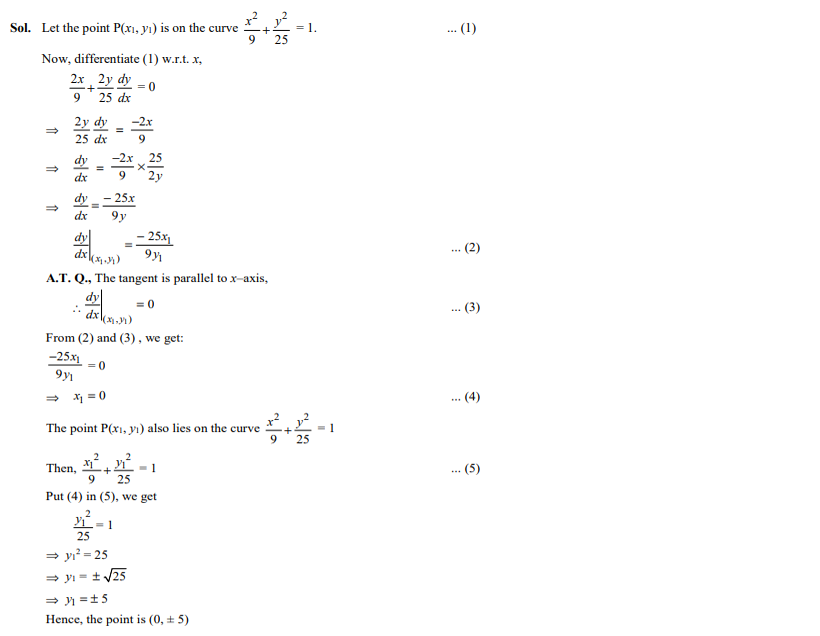## Three points are collinear, then x is equal to

1. 0
2. 2
3. 3
4. 1

Ans. (d)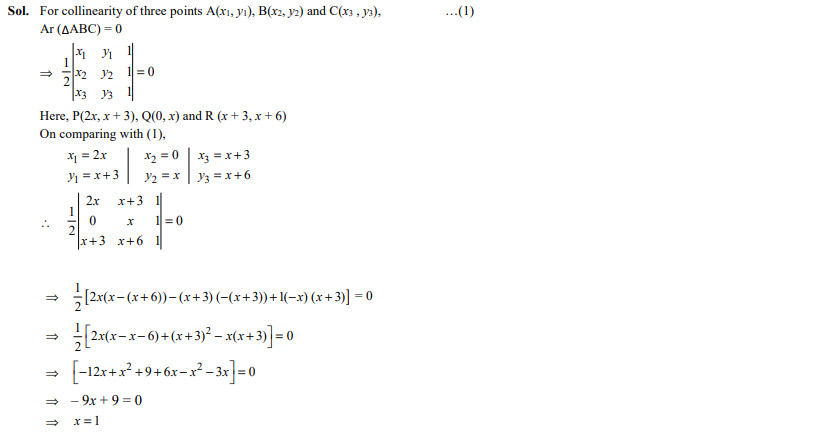## The principal value of is

1. $\frac{\mathrm{\pi }}{3}$
2. $\mathrm{\pi }$
3. $\frac{\mathrm{\pi }}{3}$
4. $\frac{\mathrm{\pi }}{6}$

Ans: (a)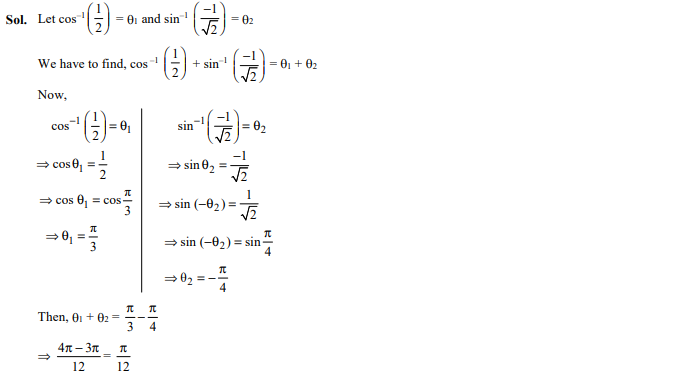## If

Ans. (c)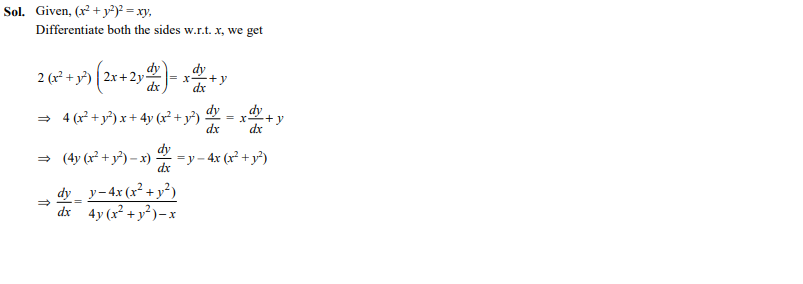## If a matrix A is both symmetric and skew symmetric, then A is necessarily a

1. Diagonal matrix
2. Zero square matrix
3. Square matrix
4. Identity matrix

Ans. (b)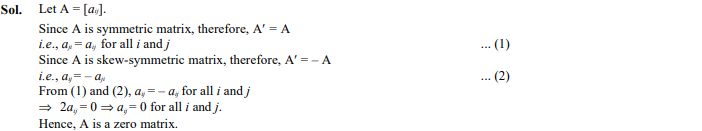## Let set X = {1, 2, 3} and a relation R is defined in X as : R = {(1, 3), (2, 2), (3, 2)}, then minimum ordered pairs which should be added in relation R to make it reflexive and symmetric are

1. {(1, 1), (2, 3), (1, 2)}
2. {(3, 3), (3, 1), (1, 2)}
3. {(1, 1), (3, 3), (3, 1), (2, 3)}
4. {(1, 1), (3, 3), (3, 1), (1, 2)}

Ans. (c)

Sol. To make R reflexive: Add (3, 1), (2, 3) in the relation R.
To make R symmetric: Add (1, 1), (3, 3) in the relation R.
The ordered pairs should be added in Relation ‘R’ are {(1, 1) (3, 3) (3, 1), (2, 3)}.

##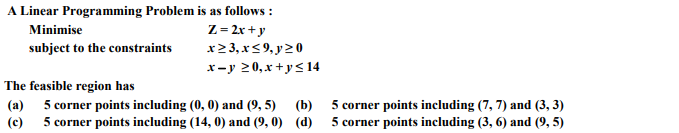Ans. (b)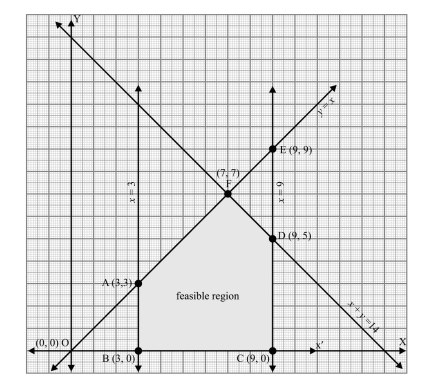## The function is continuous at x = 0 for the value of k, as

1. 3
2. 5
3. 2
4. 8

Ans.(d)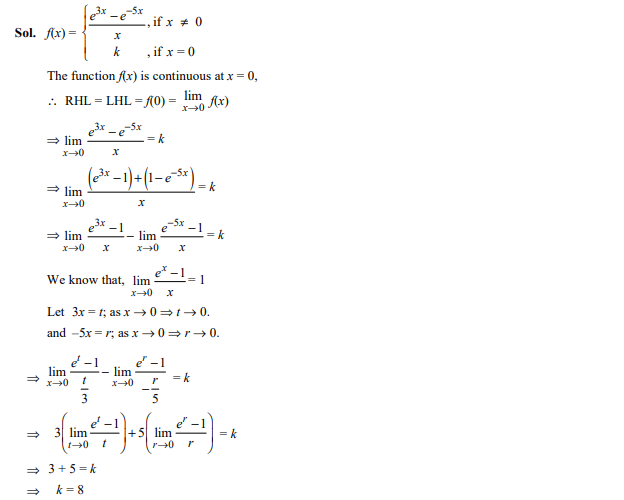## If denotes the cofactor of element of the matrix P = then the value of .

1. 5
2. 24
3. $-5$
4. $-24$

Ans.(a)

Sol. Given, matrix is

P

${C}_{23}={\left(-1\right)}^{2+3}\left|\begin{array}{cc}-1& -1\\ 3& 2\end{array}\right|=-\left(2+3\right)=-5$

Then,

## The function is decreasing in the interval is decreasing in the interval

Ans. (d)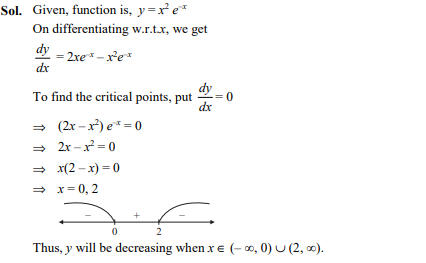## If R is a relation in set Z, then domain of R is

1. {0, 1, 2}
2. {–2, –1, 0, 1, 2}
3. {0, –1, –2}
4. {–1, 0, 1}

Ans. (b)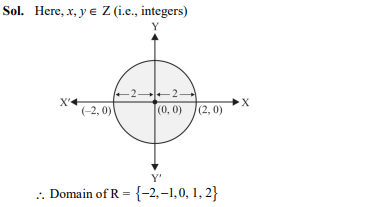## The system of linear equations 5x + ky = 5, 3x + 3y = 5; will be consistent if

1. k $\ne$ – 3
2. k = – 5
3. k = 5
4. k $\ne$ 5

Ans. (d)

Sol.
The system of linear equation will be consistent if

Now,

## The equation of the tangent to the curve where it crosses the x-axis is

1. x – 5y = 2
2. 5x – y = 2
3. x + 5y = 2
4. 5x + y = 2

Ans. (c)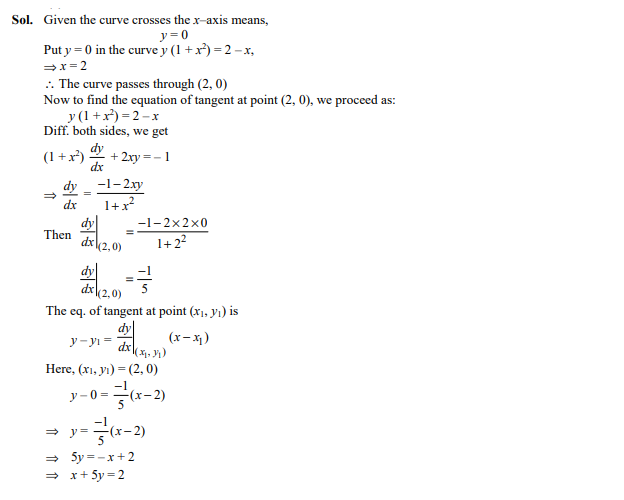## If are equal, then value of ab$-$cd is

1. 4
2. 16
3. $-$6
4. $-$16

Ans. (a)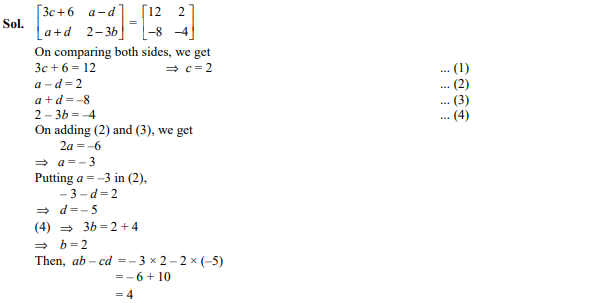## The principal value of is

1. $\frac{\mathrm{\pi }}{8}$
2. $\frac{3\mathrm{\pi }}{8}$
3. $-\frac{\mathrm{\pi }}{8}$
4. $-\frac{3\mathrm{\pi }}{8}$

Ans. (a)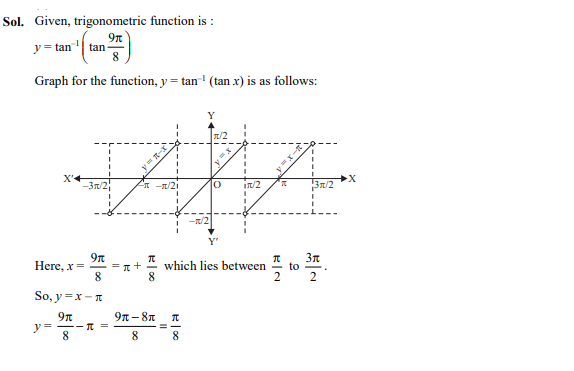## For two matrices P = and , P$-$Q is

Ans. (b)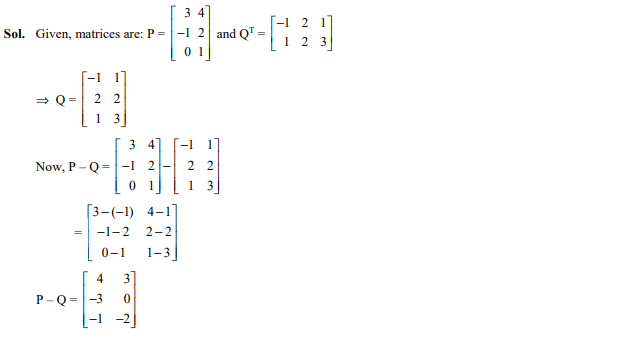SECTION-B
(In this section, there are 20 questions. Any 16 are to be attempted).

## The function is increasing in the interval1. $\left[3,\infty \right)$

Ans: (c);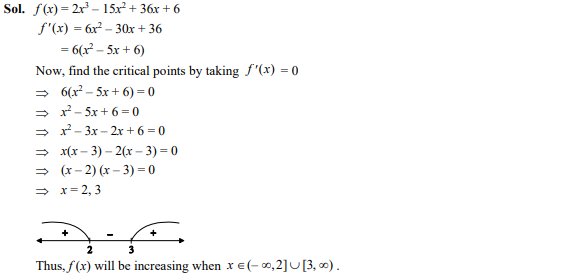## If

Ans. (b)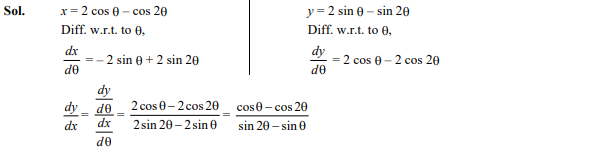## What is the domain of the function

1. $\left(1,2\right)$
2. $\left(-1,1\right)$

Ans: (d);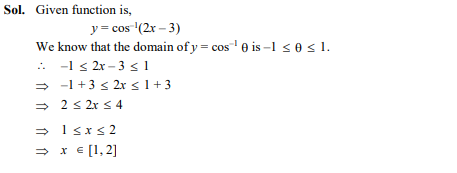##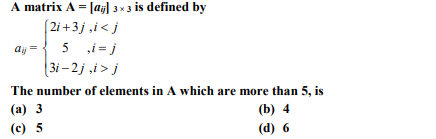Ans: (b);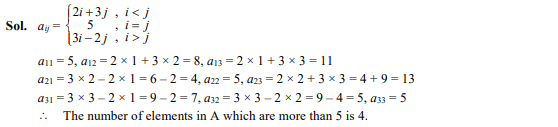## If a function f defined by , then the value of k is

1. 2
2. 3
3. 6
4. $-$6

Ans. (c)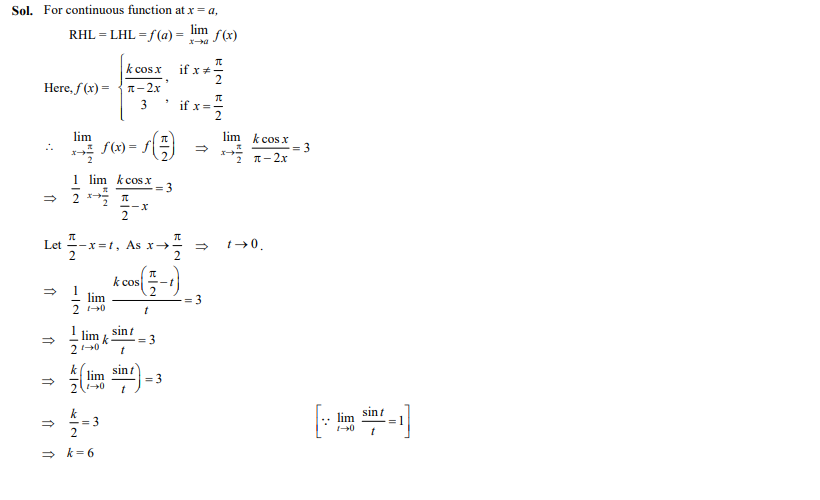## For the matrix is

1. 2I
2. 3I
3. I
4. 5I

Ans. (a)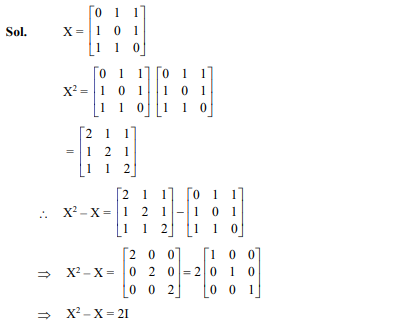## Let and the function is defined by $\mathrm{f}\left(\mathrm{x}\right)={\mathrm{x}}^{2},\mathrm{x}\in \mathrm{N}.$ Then this function is

1. injective only
2. not bijective
3. surjective only
4. bijective

Ans.(d)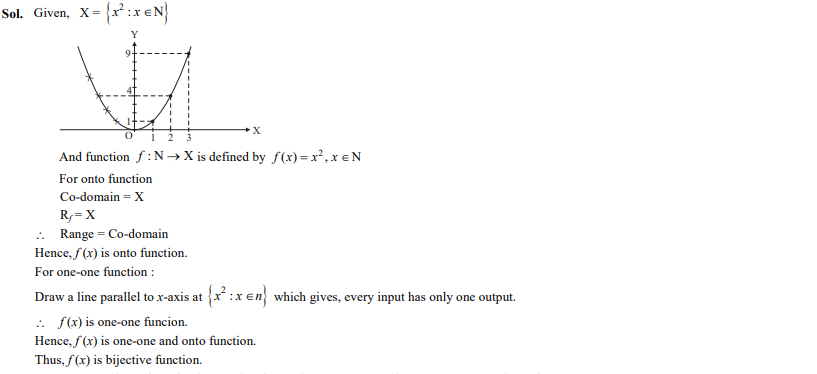## The corner points of the feasible region for a Linear Programming problem are P(0, 5), Q(1, 5), R(4, 2) and S(12, 0). The minimum value of the objective function Z = 2x + 5y is at the point

1. P
2. Q
3. R
4. S

Sol. (c);

Given, points of the feasible region: P (0, 5), Q (1, 5), R (4, 2) and S (12, 0) and objective function Z = 2x + 5y

Here,

Hence, the minimum value of Z is at the point R.

## The equation of the normal to the curve at the point

Ans. (d)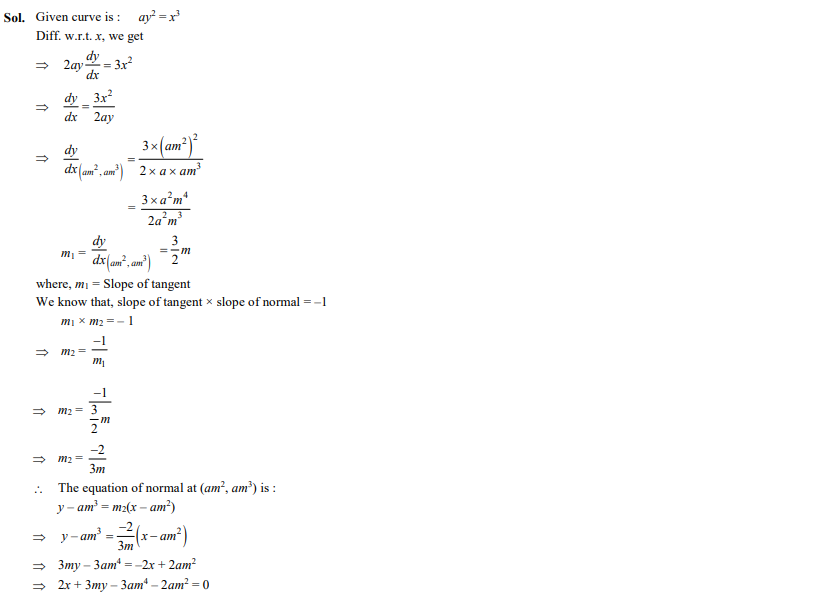## If A is a square matrix of order 3 and ,then is

1. 125
2. –25
3. 25
4. ±25

Ans. (c)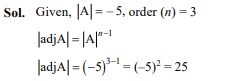## The simplest form of

1. $\frac{\mathrm{\pi }}{4}-\frac{1}{2}{\mathrm{cos}}^{-1}x$
2. $\frac{\mathrm{\pi }}{4}+\frac{1}{2}{\mathrm{cos}}^{-1}x$

Ans.(c)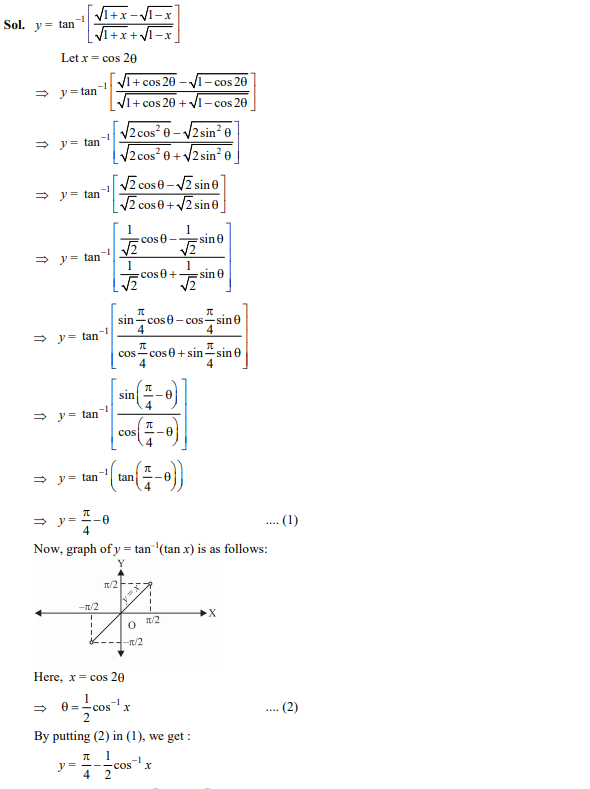## If for the matrix then the value of is

1. $±3$
2. $-3$
3. $±1$
4. $1$

Ans : (a);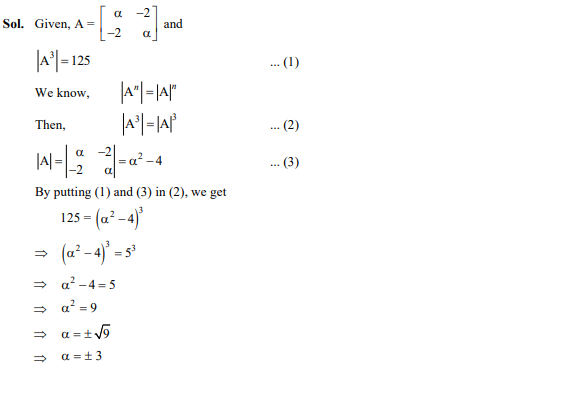## If then which one of the following equations is true?

1. $\left(1-{x}^{2}\right)\frac{{d}^{2}y}{d{x}^{2}}+x\frac{dy}{dx}+{m}^{2}y=0$
2. $\left(1-{x}^{2}\right)\frac{{d}^{2}y}{d{x}^{2}}-x\frac{dy}{dx}+{m}^{2}y=0$
3. $\left(1+{x}^{2}\right)\frac{{d}^{2}y}{d{x}^{2}}-x\frac{dy}{dx}-{m}^{2}y=0$
4. $\left(1+{x}^{2}\right)\frac{{d}^{2}y}{d{x}^{2}}+x\frac{dy}{dx}-{m}^{2}x=0$

Ans. (b)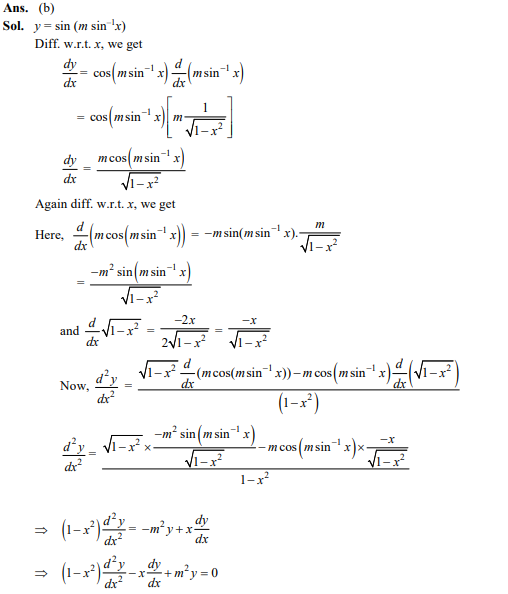## The principal value of is

1. $\mathrm{\pi }$
2. $-\frac{\mathrm{\pi }}{2}$
3. 0
4. $2\sqrt{3}$

Ans. (b)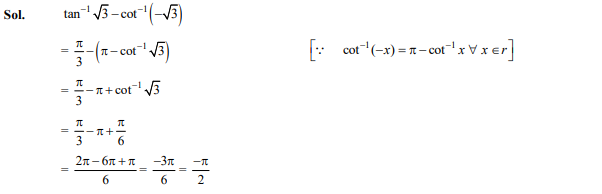## The maximum value of is

1. ${e}^{1/e}$
2. $e$
3. ${\left(\frac{1}{e}\right)}^{1/e}$
4. ${e}^{e}$

Ans. (a)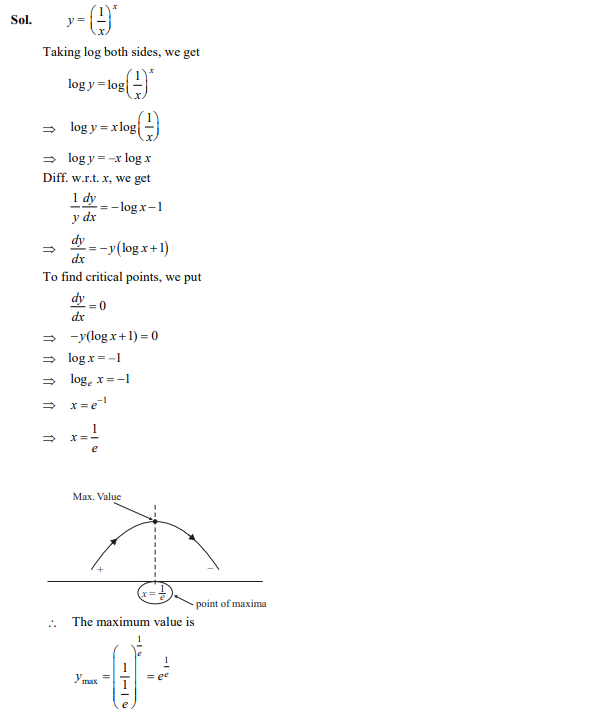## Let matrix is given by Then the matrix where = Minor of ,is

1. $\left[\begin{array}{ccc}7& -5& -3\\ 19& 1& -11\\ -11& 1& 7\end{array}\right]$
2. $\left[\begin{array}{ccc}7& -19& -11\\ 5& -1& -1\\ 3& 11& 7\end{array}\right]$
3. $\left[\begin{array}{ccc}7& 19& -11\\ -3& 11& 7\\ -5& -1& -1\end{array}\right]$
4. $\left[\begin{array}{ccc}7& 19& -11\\ -1& -1& 7\\ -3& -11& 7\end{array}\right]$

Ans. (d)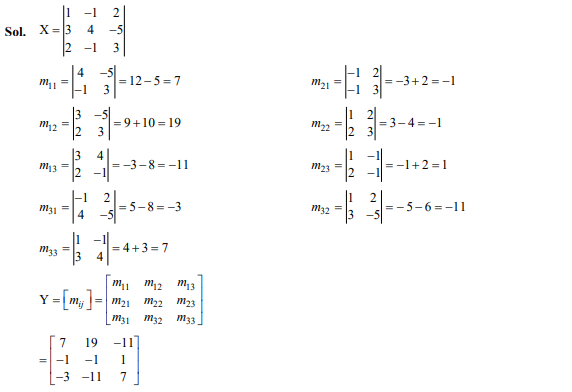## A function defined by

1. not one-one
2. one-one
3. not onto
4. neither one-one nor onto

Ans. (d)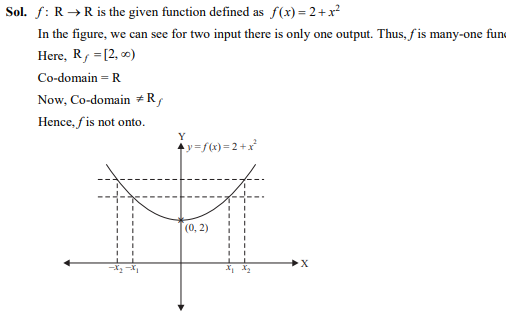##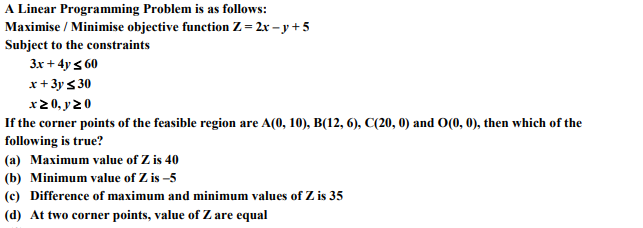Ans. (b)

Sol: Objective function Z = 2x – y + 5

Points of the feasible region are
A(0, 10), B(12, 6), C(20, 0) and O(0, 0)

## If x = – 4 is a root of then the sum of the other two roots is

1. 4
2. -3
3. 2
4. 5

Ans. (a)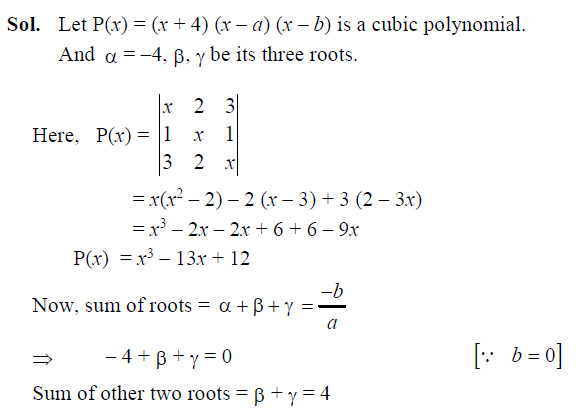## The absolute maximum value of the function in the interval is

1. 8
2. 9
3. 6
4. 10

Ans. (a)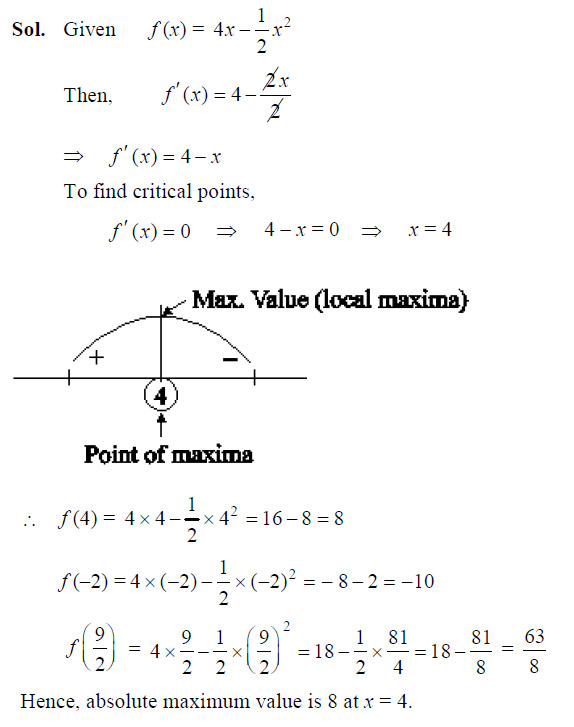SECTION-C
(Attempt any 8 questions out of the Questions 41–50. Each question is of one mark).

## In a sphere of radius r, a right circular cone of height h having maximum curved surface area is inscribed. The expression for the square of curved surface of cone is

Ans. (c)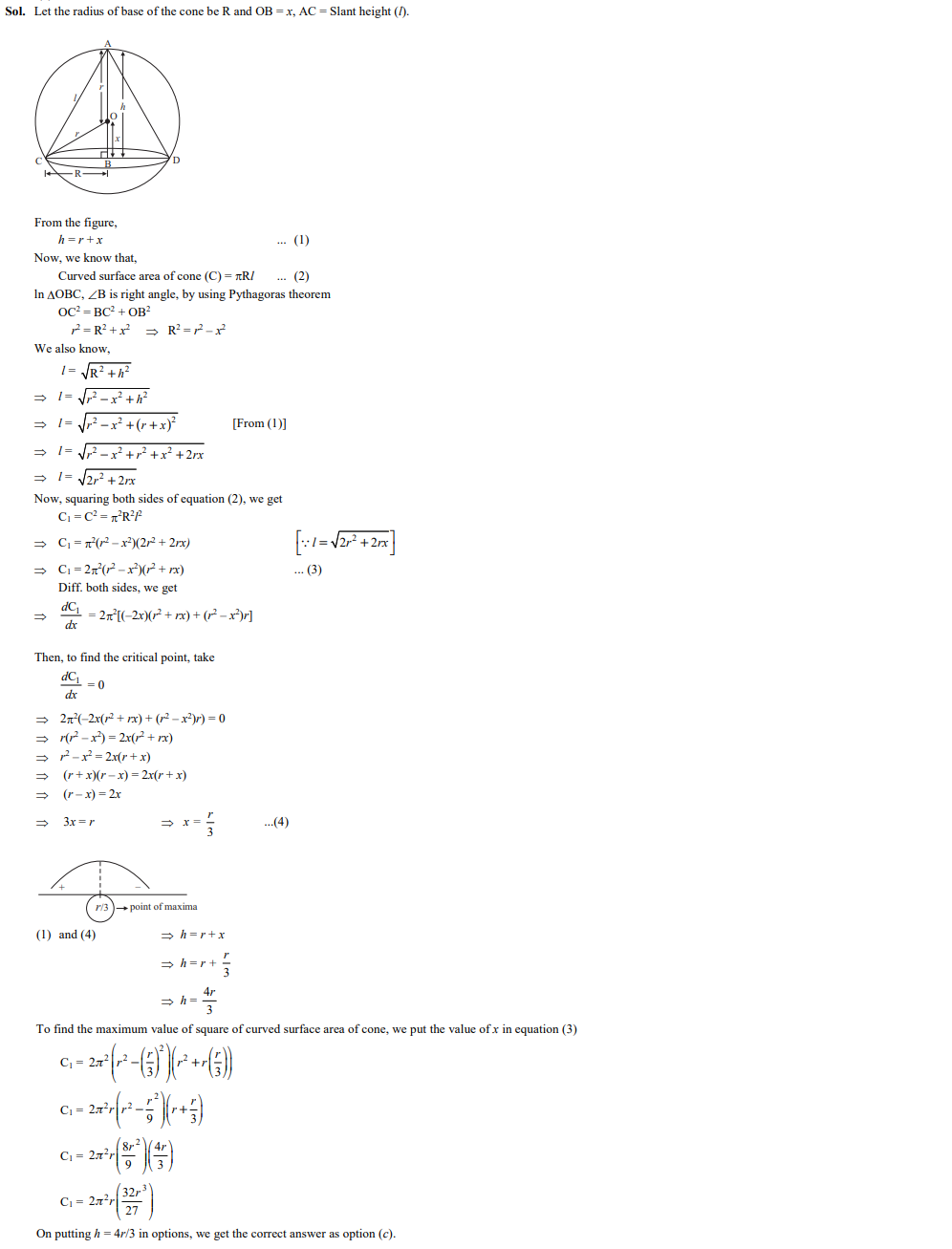## The corner points of the feasible region determined by a set of constraints (linear inequalities) are P(0, 5), Q(3, 5), R(5, 0) and S(4, 1) and the objective function is Z = ax + 2by where a, b > 0. The condition on a and b such that the maximum Z occurs at Q and S is

1. a – 5b = 0
2. a – 3b = 0
3. a – 2b = 0
4. a – 8b = 0

Ans. (d)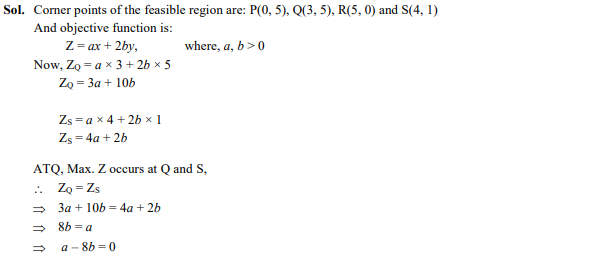## If curves and xy = c cut at right angles, then the value of c is

1. $4\sqrt{2}$
2. $8$
3. $2\sqrt{2}$
4. $-4\sqrt{2}$

Ans. (a)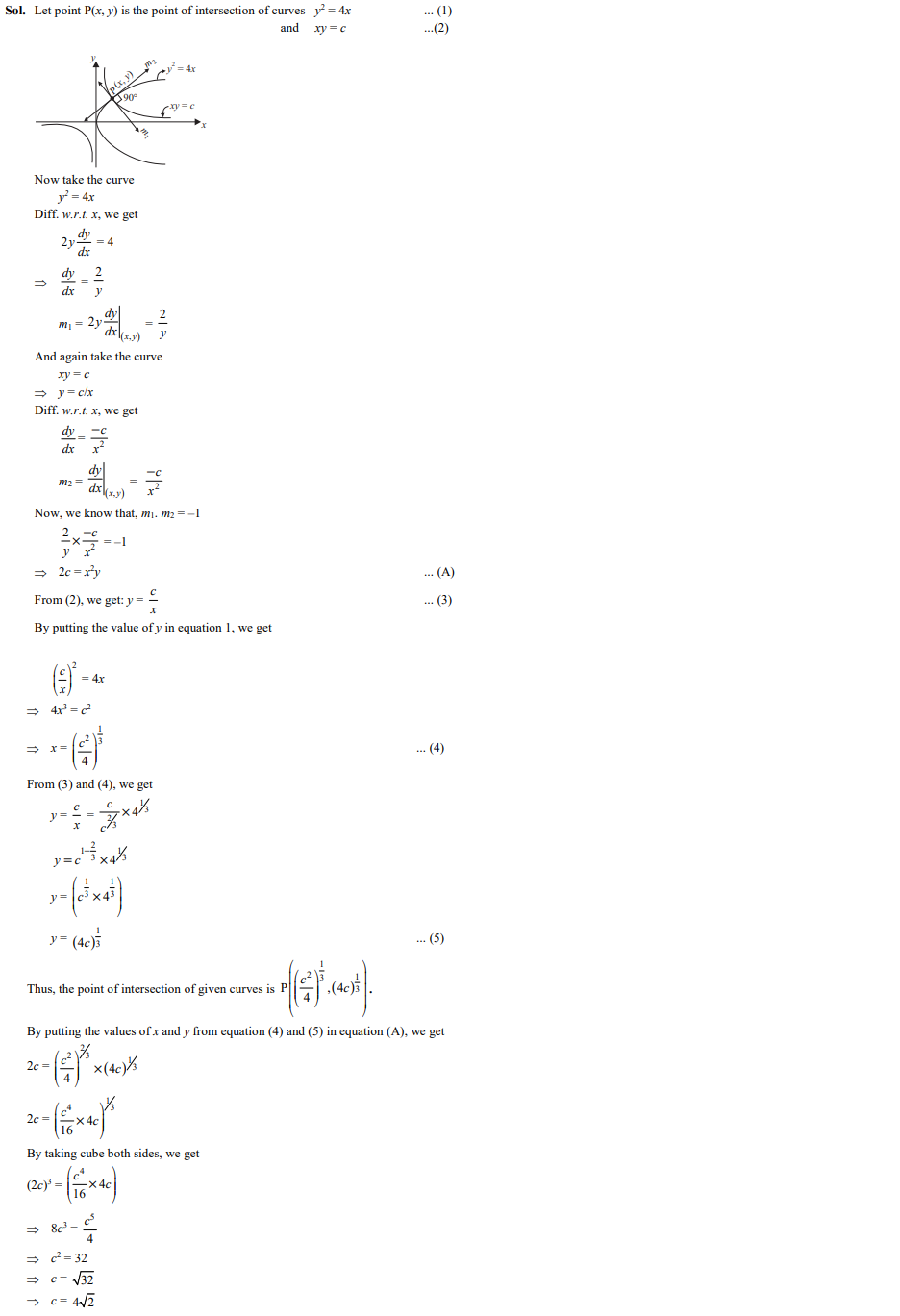## The inverse of the matrix is

Ans. (d)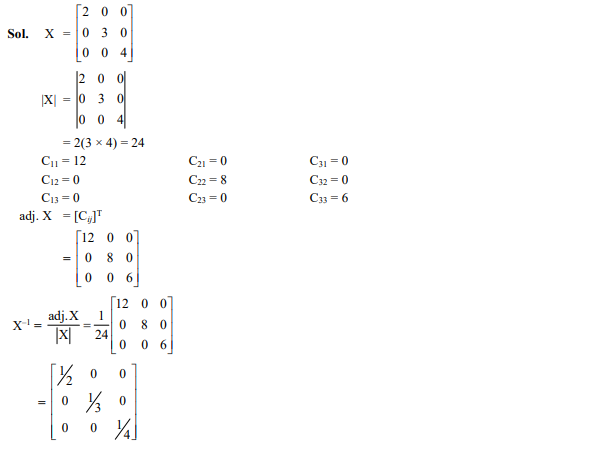## For an L.P.P. the objective function is Z = 4x + 3y, and the feasible region determined by a set of constraints (linear inequations) is shown in the graph.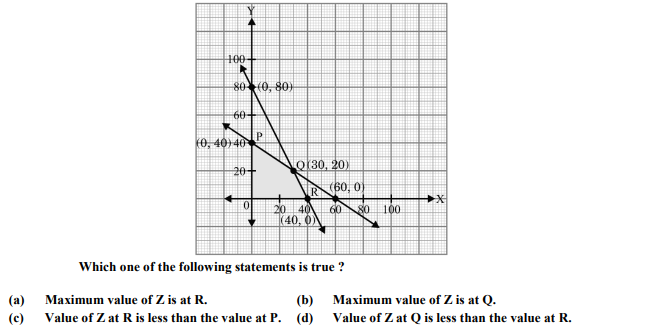Ans. (b)

Sol. Z = 4x + 3y

Corner points of feasible region are P(0, 40), O(0, 0), R(40, 0), and Q(30, 20)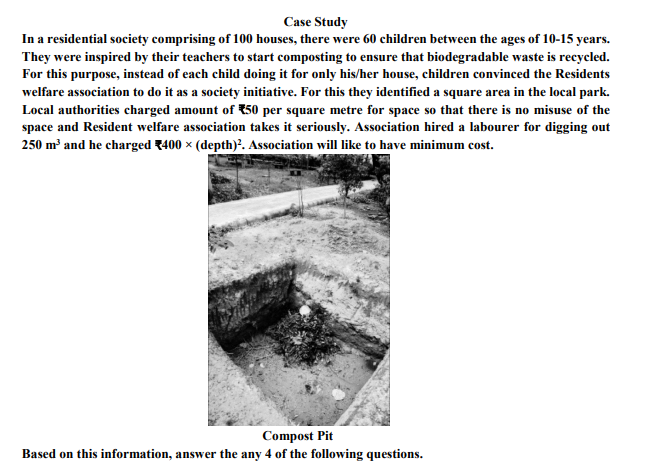## Let side of square plot is x m and its depth is h metres, then cost c for the pit is

1. $\frac{50}{h}+400{h}^{2}$
2. $\frac{12500}{h}+400{h}^{2}$
3. $\frac{250}{h}+{h}^{2}$
4. $\frac{250}{h}+400{h}^{2}$

Ans. (b)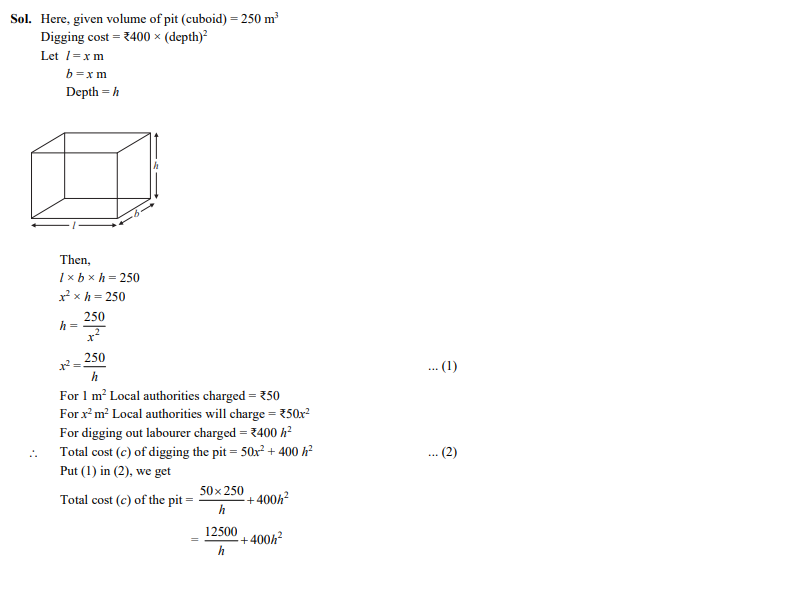## Value of h (in m) for which is

1. 1.5
2. 2
3. 2.5
4. 3

Ans. (c)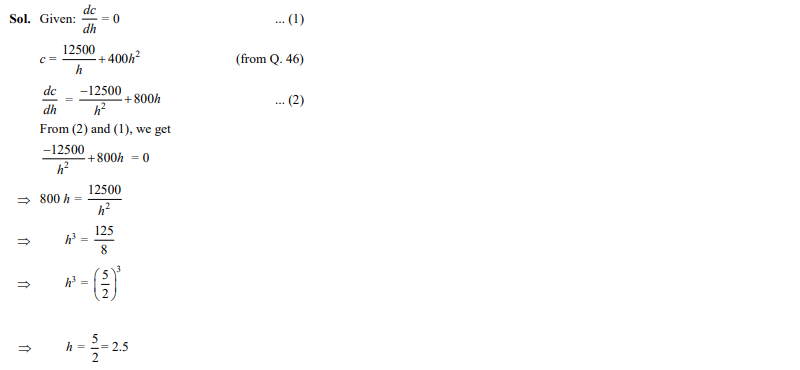## is given by

1. $\frac{25000}{{h}^{3}}+800$
2. $\frac{500}{{h}^{3}}+800$
3. $\frac{100}{{h}^{3}}+800$
4. $\frac{500}{{h}^{3}}+2$

Ans. (a)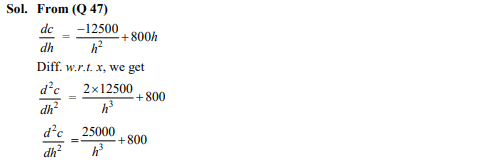## Value of x (in m) for minimum cost is

1. 5
2. $10\sqrt{\frac{5}{3}}$
3. $5\sqrt{5}$
4. $10$

Ans. (d)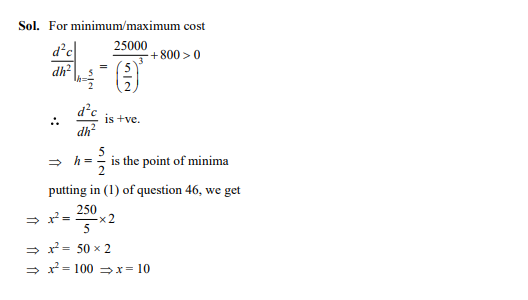## Total minimum cost of digging the pit (in ₹) is

1. 4,100
2. 7,500
3. 7,850
4. 3,220

Ans. (b)